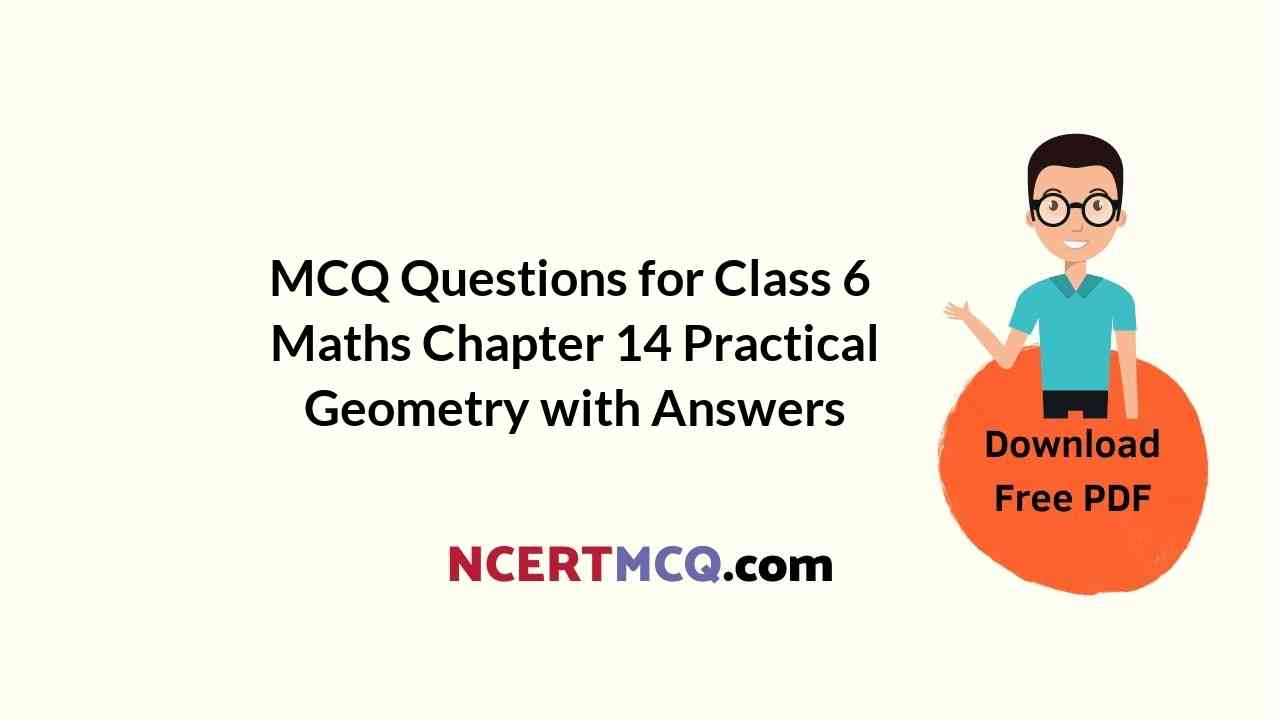Check the below NCERT MCQ Questions for Class 6 Maths Chapter 14 Practical Geometry with Answers Pdf free download. MCQ Questions for Class 6 Maths with Answers were prepared based on the latest exam pattern. We have provided Practical Geometry Class 6 Maths MCQs Questions with Answers to help students understand the concept very well.

Students can also refer to NCERT Solutions for Class 6 Maths Chapter 14 Practical Geometry for better exam preparation and score more marks.

## Practical Geometry Class 6 MCQs Questions with Answers

Practical Geometry Class 6 MCQ Question 1.
A line segment $$\overrightarrow { TP }$$ is bisected at I. What is the measure of $$\overrightarrow { TI }$$?
(a) $$\frac{1}{2}$$$$\overrightarrow { TP }$$
(b) $$\overrightarrow { IP }$$
(c) $$\overrightarrow { TP }$$
(d) $$\frac{1}{3}$$ $$\overrightarrow { TP }$$

Answer: (b) $$\overrightarrow { IP }$$
Hint:
$$\overrightarrow { TI }$$ = $$\frac{1}{3}$$ $$\overrightarrow { TP }$$ = IP

MCQ On Practical Geometry Class 6 Question 2.
Which of the following can be drawn on a piece of paper?
(a) A line
(b) A line segment
(c) A ray
(d) A plane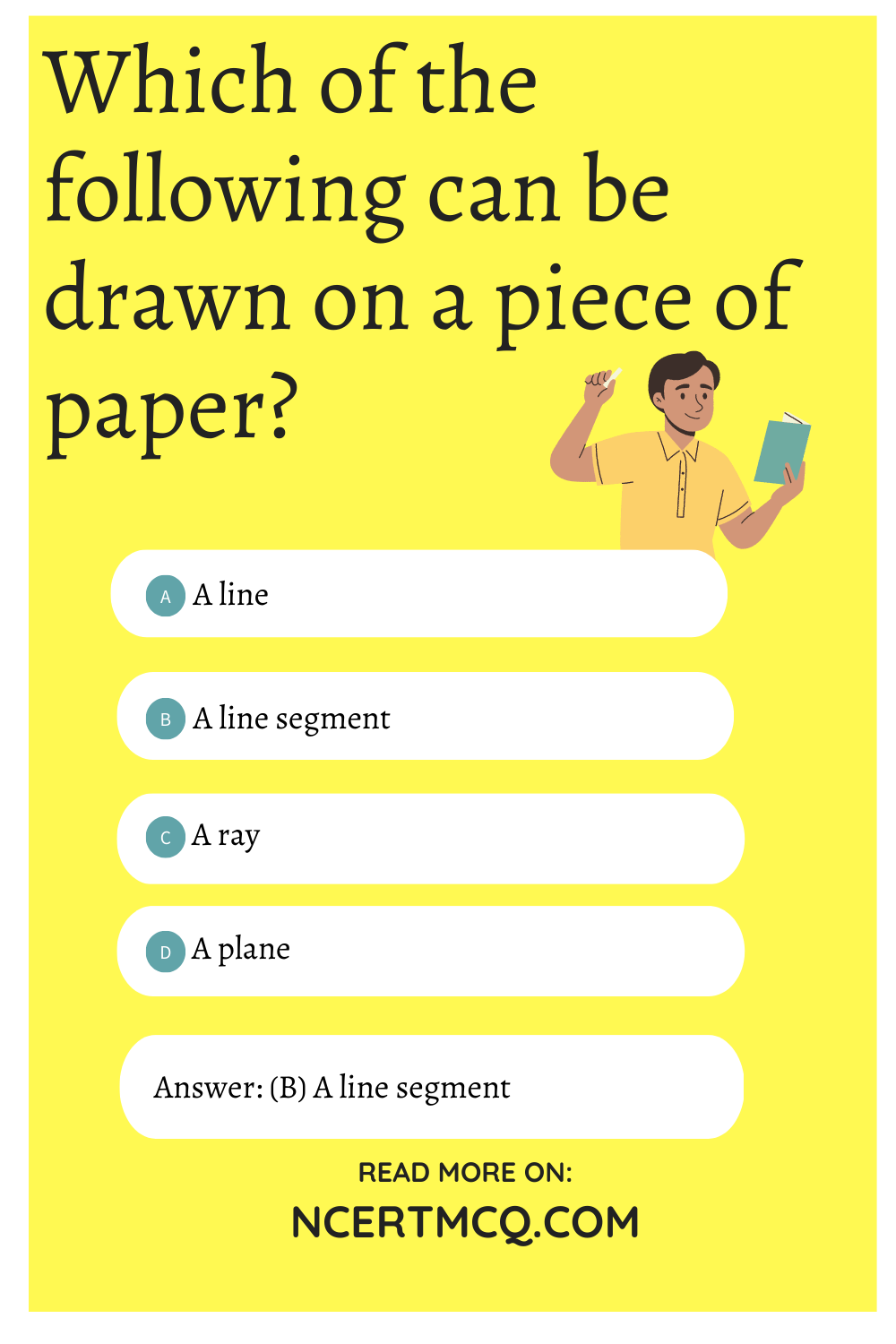MCQ Questions For Class 6 Maths Chapter 14 Question 3.
l || m. P and Q are points on land m respectively such that PQ ⊥ lR is a point on a line n in the same plane such that $$\overline { PQ }$$ = $$\overline { QR }$$. Which of the following is true?
(a) l || n
(b) m || n
(c) Both [a] and [b]
(d) Neither [a] nor [b]

Answer: (c) Both [a] and [b]
Hint: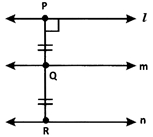Clearly, from the given data and the figure, l || n and m || n.

Class 6 Maths Chapter 14 MCQ Question 4.
At 7 a.m. the angle between the Sun’s ray and the ground at a point is 43°. What would be the angle at 10 a.m.?
(a) 40°
(b) 90°
(c) Between 43° and 90°
(d) Greater than 90°

Answer: (c) Between 43° and 90°
Hint:Let QP be the sun’s ray and RP be the ground. The angle between QP and PR at P is 43° at 7 a.m. At 10 a.m., the sun’s ray is Q’P. We know that at 12 noon the sun is exactly above our head. So, the sun’s ray will be perpendicular to the ground. So, clearly at 10 am, the required angle will be between 43° and 90°.

MCQ On Practical Geometry For Class 6 Question 5.
$$\overrightarrow { XY }$$ divides ∠MXN = 72° in the ratio 1 : 2. What is the measure of ∠YXN?(a) 48°
(b) 24°
(c) 72°
(d) 96°

Hint: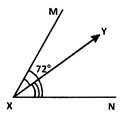Given ∠MXN = 72∘ and $$\overrightarrow { XY }$$ divides ∠MXN in the ratio 1 : 2.
∠YXN = $$\frac{2}{3}$$ ∠MXN = $$\frac{2}{3}$$ × 72° = 48°

MCQ Of Practical Geometry Class 6 Question 6.
$$\overrightarrow { MN }$$ is the perpendicular bisector of $$\overleftrightarrow { AB }$$. Which of the given statements is correct?
(i) ∠ANM + ∠MNB = 90∘
(ii) $$\overline { AN }$$ = $$\overline { NB }$$
(iii) $$\overline { AN }$$ = 2 $$\overline { NB }$$
(iv) ∠MNB = $$\frac{1}{2}$$ ∠ANM
(a) (i) and (iii) only
(b) (ii) and (iv) only
(c) (i) and (ii) only
(d) (ii) and (iii) only

Answer: (c) (i) and (ii) only
Hint:
$$\overrightarrow { NM }$$ ⊥ $$\overleftrightarrow { AB }$$ and $$\overrightarrow { NM }$$ divides $$\overleftrightarrow { AB }$$ into two congruent parts.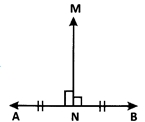Clearly ∠ANM = ∠MNB = 90∘ is true. $$\overline { AN }$$ = $$\overline { NB }$$ is true since $$\overrightarrow { NM }$$ ⊥ $$\overleftrightarrow { AB }$$, $$\overline { AN }$$ = 2$$\overline { NB }$$ is false, and ∠MNB = $$\frac{1}{2}$$ ∠ANM is false. Thus, only (i) and (ii) are correct.

Class 6 Maths Practical Geometry MCQ Question 7
Identify the uses of a ruler.
(a) To draw a line segment of a given length
(b) To draw a copy of a given segment.
(c) To draw a diameter of a circle.
(d) All the above.

Hint:
A ruler is used to draw a line segment of a given length, to draw the copy of a given segment, and to draw a diameter of a circle. Thus, all the given options are correct.

Practical Geometry MCQ Class 6 Question 8.
P is the midpoint of $$\overline { AB }$$. M and N are midpoints of $$\overline { AP }$$ respectively. What is the measure of $$\overline { MN }$$?
(a) $$\frac{1}{3}$$ $$\overline { AB }$$
(b) $$\frac{1}{2}$$ $$\overline { AB }$$
(c) $$\frac{1}{2}$$ $$\overline { AP }$$
(d) $$\frac{3}{2}$$ $$\overline { AB }$$

Answer: (b) $$\frac{1}{2}$$ $$\overline { AB }$$
Hint: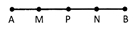P is the midpoint of $$\overline { AB }$$ ⇒ AP = PB M and N are midpoints of $$\overline { AP }$$ and $$\overline { PB }$$ ⇒ $$\overline { AM }$$ = $$\overline { MP }$$ and $$\overline { PN }$$ = $$\overline { NB }$$
∴ $$\overline { MN }$$ = $$\overline { MP }$$ + $$\overline { PN }$$ = $$\frac{1}{2}$$ $$\overline { AP }$$ + $$\frac{1}{2}$$ $$\overline { PB }$$ = $$\frac{1}{2}$$ ($$\overline { AP }$$ + $$\overline { PB }$$ ) = $$\frac{1}{2}$$ ($$\overline { AB }$$)

Class 6 Practical Geometry MCQ Question 9.
$$\overrightarrow { XY }$$ bisects∠AXB. If ∠YXB = 37.5∘, what is the measure of ∠AXB?
(a) 37.5°
(b) 74°
(c) 64°
(d) 75°

MCQ Practical Geometry Class 6 Question 10.
X and Y are two distinct points in a plane. How many lines can be drawn passing through both X and Y?
(a) 0
(b) 1
(c) Only 2
(d) Infinitely many

Ncert Class 6 Maths Chapter 14 MCQ Question 11.
Lines a, b, p, q, m, n and x have a point P common to all of them. What is the name of P?
(a) Point of concurrence
(b) Point of intersection
(c) Common point
(d) Distinct point

Hint:
A point common to multiple lines is called a point of concurrence as the lines are concurrent lines.

Class 6 Maths Chapter 14 Extra Questions Question 12.
$$\overrightarrow { QZ }$$ is the bisector of ∠PQZ = ∠PQR. Which of the following is true?
(a) ∠PQZ = ∠PQR
(b) ∠PQZ = ∠ZQR
(c) ∠PQZ = $$\frac{1}{2}$$ ∠ZQR
(d) Both [b] and [c]

Answer: (d) Both [b] and [c]
Hint:
$$\overrightarrow { QZ }$$ bisects ∠PQR (Given)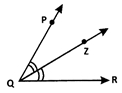Thus. ∠PQZ = ∠ZQR = $$\frac{1}{2}$$ ∠PQR

MCQ On Lines And Angles For Class 6 Question 13.
Identify the pair of parallel lines.
(i) Lines m and n have two points in common.
(ii) Lines p and q do not have any point in common
(iii) Lines p and q have a point X in common.
(a) (i) and (ii) only
(b) (ii) only
(c) (ii) and (iii) only
(d) (i), (ii) and (iii)

Hint:
Parallel lines do not have any point in common.

Class 6 Maths Ch 14 MCQ Question 14.
Identify the one with no definite length.
(a) $$\overleftrightarrow { AB }$$
(b) $$\overline { PQ }$$
(c) -XYZ
(d) $$\overline { MN }$$

Answer: (a) $$\overleftrightarrow { AB }$$
Hint:
$$\overleftrightarrow { AB }$$ has no definite length.

Question 15.
If two lines have only one point in common, what are they called?
(a) Parallel lines
(b) Intersecting lines
(c) Perpendicular lines
(d) Transversal

Hint:
Intersecting lines have only one point in common.

Question 16.
Two lines are said to be perpendicular to each other when they meet at ____angle.
(a) 180°
(b) 90°
(c) 60°
(d) 360°

Question 17.
A line segment $$\overline { IP }$$ is bisected at T. Which of the following equals $$\overline { IT }$$?
(a) $$\overline { IP }$$
(b) $$\overline { TP }$$
(c) $$\overline { TC }$$
(d) $$\overline { IQ }$$

Answer: (b) $$\overline { TP }$$

Question 18.
How do you draw a 90° angle?
(a) By drawing a perpendicular to a line from a point lying on it.
(b) By bisecting a 120° angle.
(c) By bisecting a 60° angle.
(d) By drawing multiples of 45° angle.

Answer: (a) By drawing a perpendicular to a line from a point lying on it.

Question 19.
An angle of 15° is drawn using a pair of compasses and a ruler. How is it done?
(a) Bisecting 60° angle.
(b) Bisecting 60° and 120° angles.
(c) Bisecting 60° and then bisecting it again.
(d) Bisecting a 60° and 180° angles.

Answer: (c) Bisecting 60° and then bisecting it again.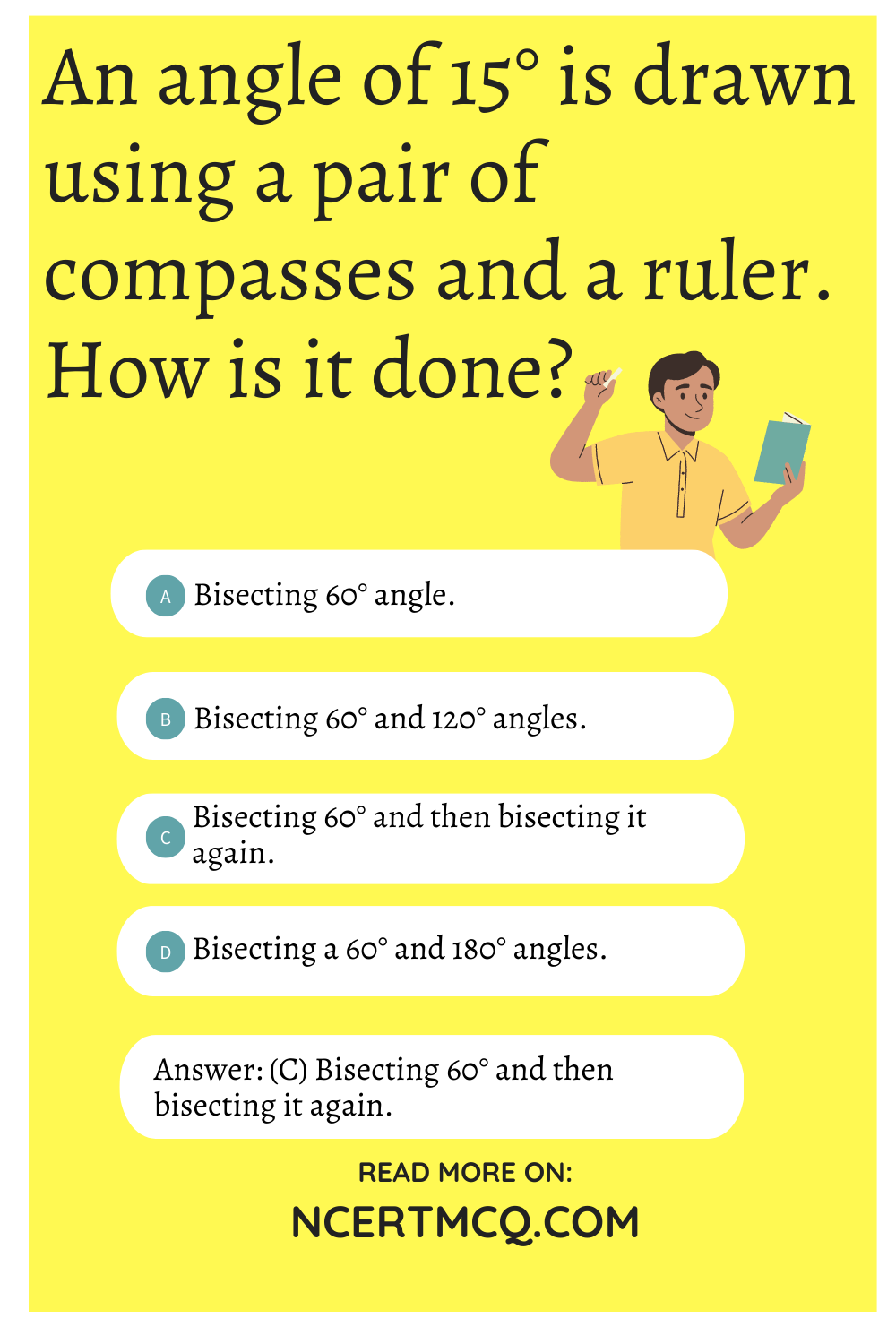Question 20.
Identify the instruments used to bisect a given line segment.
(a) A scale and a protractor
(b) Scale and compasses
(c) Scale and setsquares
(d) A scale